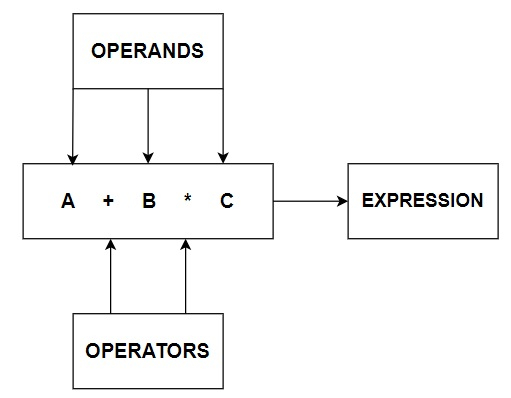# What is dot operator in Java?

### OPERATORS

In computer programming we often need to perform some arithmetical or logical operations. In such circumstances, we need operators to perform these tasks. Thus, an Operator is basically a symbol or token, which performs arithmetical or logical operations and gives us meaningful result. The values involved in the operation are called Operands.

Here is a basic Pictorial representation of Operators.Now, let us discuss the types of Operators available.

### TYPES OF OPERATORS

There are three types of Operators in Java which are −

• Arithmetical Operators

• Relational Operators

• Logical Operators

Except for these operators, there are some other operators available in Java such as Dot Operator, Scope Resolution operator, instanceOf operator, etc.

## Dot Operator

Dot operator is a syntactic element, i.e. it denotes the separation between class and package, method and class, variable and reference variable. It can also be called as separator operator. It is mainly used for separating a variable and method from a reference variable, for accessing class and sub-packages from a package, for accessing any member of class or package.

### Example

public class DotExample {  // class declaration
void calculate() {  // function to calculate area of circle
double pi = 3.14; // initializing value of pi in variable pi
int area = ((int)pi) * radius * radius; // calculating area of circle
System.out.println("Area of circle is"  + area + "square unit"); // Displaying area of circle
}
public static void main(String args[]) {  // main function declaration
DotExample obj = new DotExample(); // object of class DotExample created
obj.calculate(); // function calling using object of class DotExample, see dot operator is separating the object from the class function
}
}


### Output

Area of circle is 75 square unit


Updated on: 05-Sep-2022

4K+ Views